# Voronoi from Picture

Friday, May 22, 2015, 02:43 AM

Days ago I’ve seen this challenge on Code Golf. It ask for a Voronoi diagram that resemble the original picture as close as possible.

It hooked me on. And other’s results are great! Like a fine Divisionism/Pointillism movement. So I give it a try myself also.

import random
from PIL import Image
from PIL.ImageFilter import FIND_EDGES, GaussianBlur, SHARPEN

def coordinate(width, height):
yield from ((x, y) for y in range(height) for x in range(width))

def normalized(choices):
lower = min(weight for _, weight in choices)
upper = max(weight for _, weight in choices)
norm = lambda weight: (weight-lower) + (upper-lower)//4
return [((x, y), norm(weight)) for (x, y), weight in choices]

def random_by_weight(choices):
rand_val = random.uniform(0, sum(weight for _, weight in choices))
index = 0
count = 0
while count < rand_val:
count += choices[index]
index += 1
return choices.pop(index-1)

def init_centroids(image, cells):
width, height = image.size
edge_img = image.filter(FIND_EDGES).filter(GaussianBlur).filter(SHARPEN)
weight = lambda x, y: 256 - max(edge_img.getpixel((x, y)))
choices = [((x, y), weight(x, y)) for x, y in coordinate(width, height)]
return [random_by_weight(normalized(choices)) for _ in range(cells)]

def init_rgbs(image, centroids):
rgb_im = image.convert('RGB')
return [rgb_im.getpixel((x, y)) for x, y in centroids]

def simulate_voronoi(image_path, cells=25, scale=None):
image = Image.open(image_path)
if scale is not None:
image.thumbnail((scale, scale), Image.ANTIALIAS)
centroids = init_centroids(image, cells)
rgbs = init_rgbs(image, centroids)
return image.size, list(zip(centroids, rgbs))


Here are my results (at 500 Voronoi sites).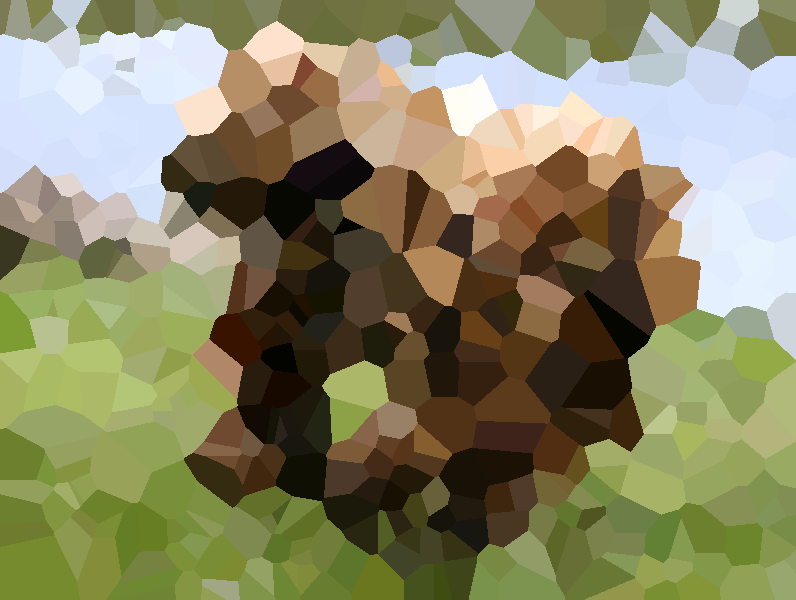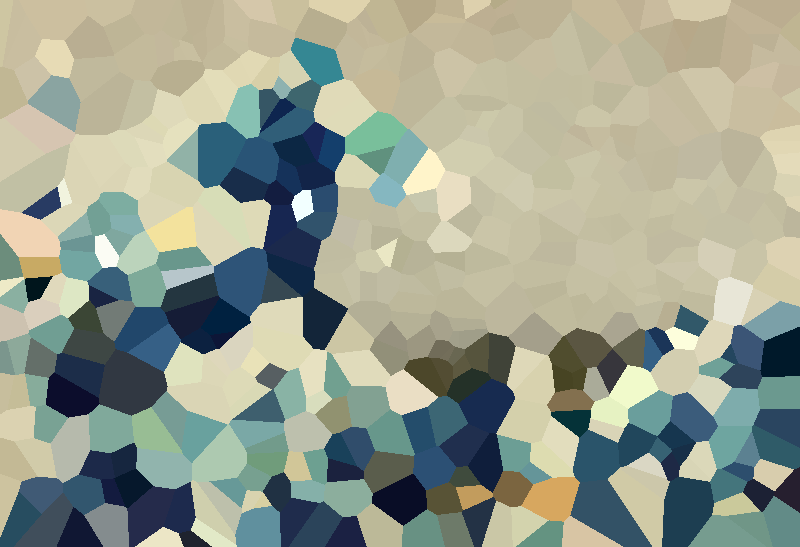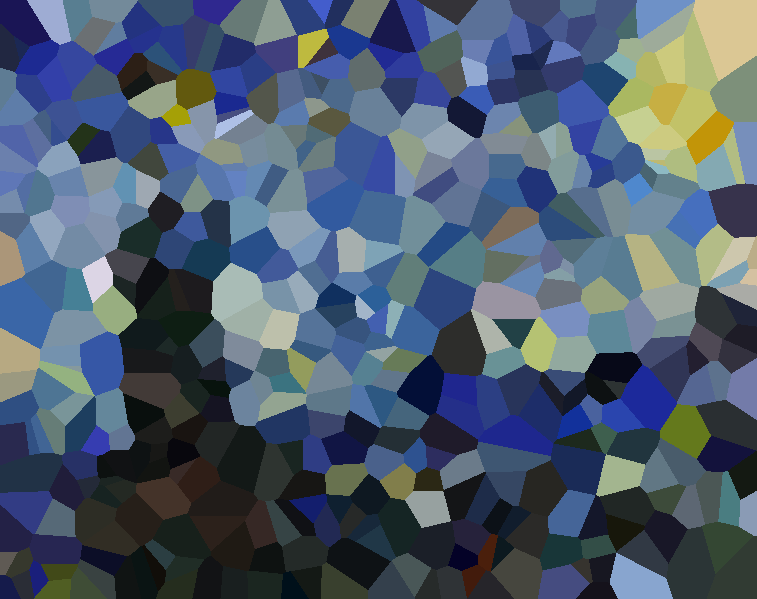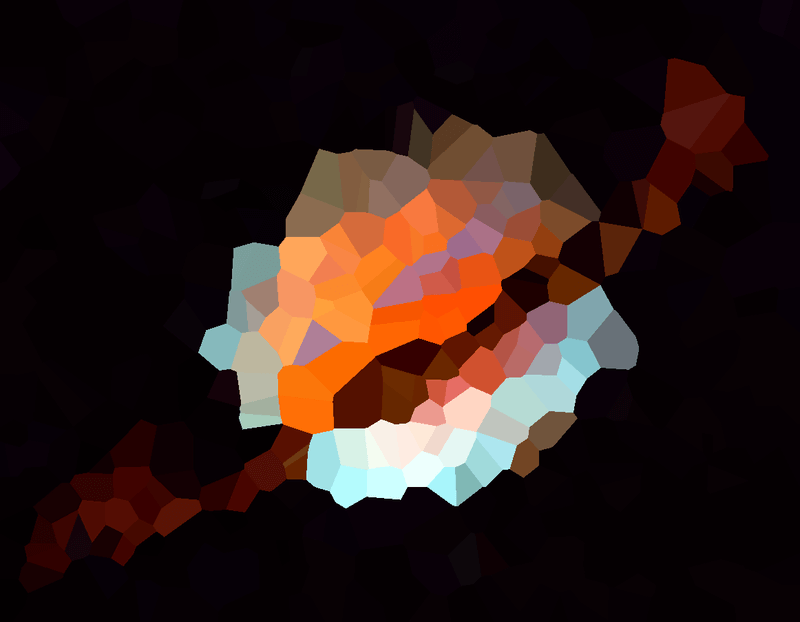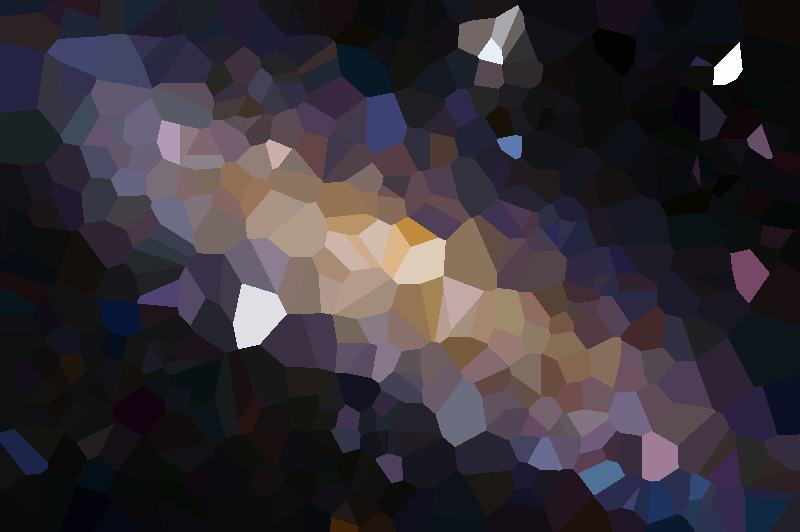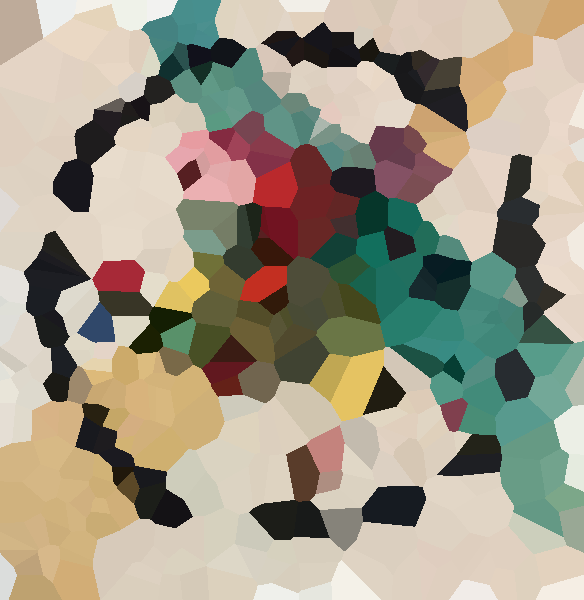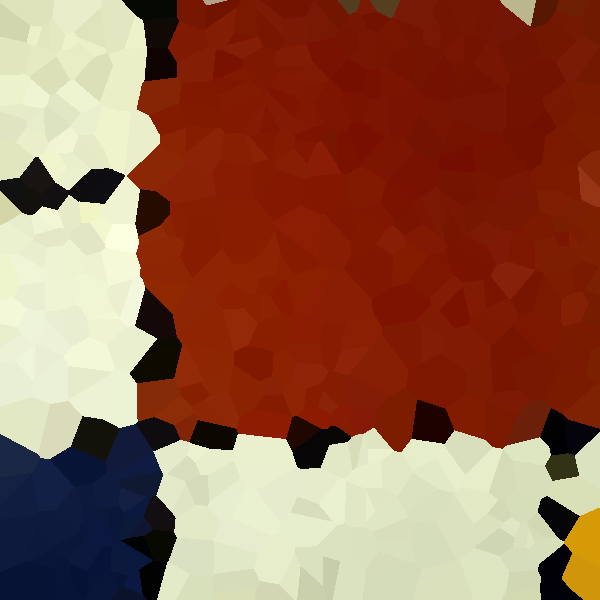Can’t say that I can compete with these. Especially consider that I have no background knowledge in image processing at all. Still, it’s a fun experience that let me try PIL for the first time.

author